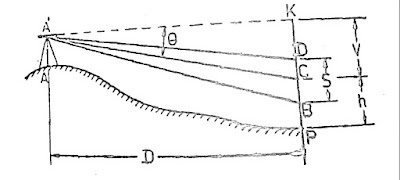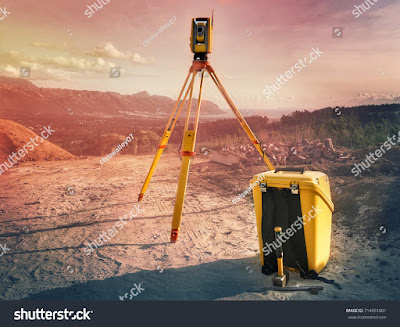How to find difference in elevation between two points.

# How to find difference in elevation between two points.

www.technoupendra.comTechno Upendra

The determine the difference of elevation between two points is an art. This work is also called levelling or measurement in the ventricle plane. For calculation of elevation also used some mathematical or trigonometric formulas. Some definition used to understand about levelling see also:- https://www.technoupendra.com/2020/01/what-is-benchmark-in-surveying.html?m=1

Instrument required to determine the difference of elevation between two points generally used.
A. Levell instrument.
B. Theodolite or Techeometre
C. Total station.
D.DGPS.

A. Levell instrument - In this method there are two methods used for finding elevation.
I. Simple levelling  II. Differential levelling

I.Simple levelling

In the above picture, the level instrument Is set between points A and B.
The staff reading A is 2.340 and staff reading B is 3.135
Then differences of level = 3.135-2.340 =0.795 m.
OR.
If the RL of A is 100
HI of at O= 100+2.340 =102.340
RL. of B =102.340-3.135 =99.205 m.

II. Differential levelling  - This method is used
i. if they are too far apart.
ii If the difference in elevation between them is too great.
iii. If there are obstacle intervening.

B. Tachometer:- Tachometer is a transit theodolite fitted stadia diagram in the telescope.Technometrics survey

Where f/i = multiplying constant
S= Staff intercept

C. Total station:- Total station also gives a reduced level of the point. Total station has the
arrangement for giving remote elevation between two points.Total stationDGPS
www.technoupendra.com